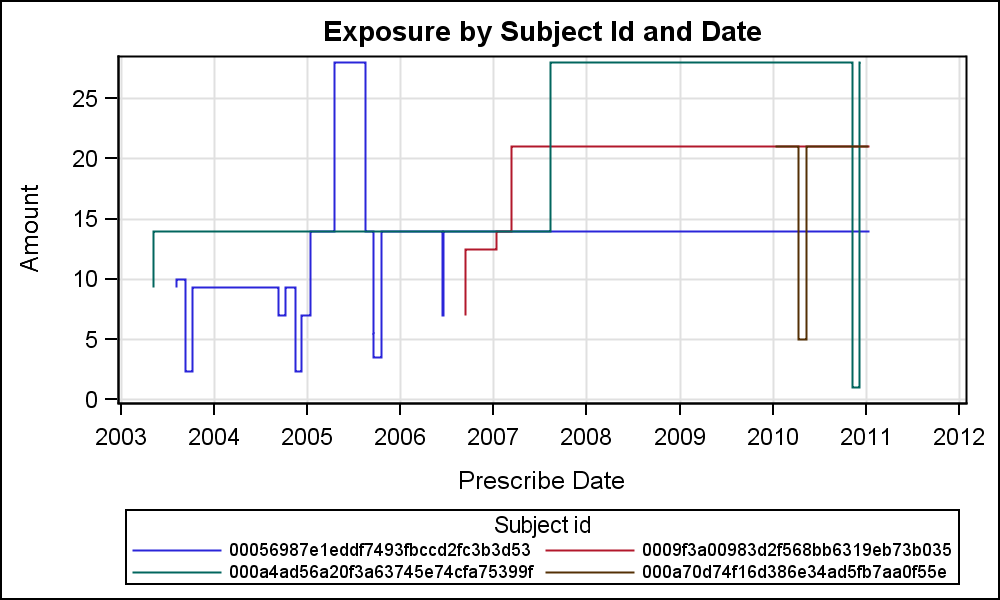## Plot time-vary drug exposure

Because both the dose and duration of drug vary between subjects and within subjects over time

I use the time-depedent Cox regression to analyze it

However, how do I explain the consistence of drug use, that a user always a user or sometimes discontinue

I consider use of figure to explain how the dose and duration vary within individual, like the figure show as belowFor example of my data and full data in the attached file. How do the sas plot the figure?  Thanks you very much

12000.1.1.7101

1

2000.1.15271514
12000.2.12142042
12000.2.27281557
12000.4.1281585
1 ACCEPTED SOLUTION

Accepted SolutionsJay54
Meteorite | Level 14

## Re: Plot time-vary drug exposureods graphics / reset attrpriority=color width=5in height=3in imagename='ExposurePlot';

title "Exposure by Subject Id and Date";

proc sgplot data=exposure2;

label id='Subject id';

label prescribe_date='Prescribe Date';

label total_amt='Amount';

step x=prescribe_date y=total_amt / group=id justify=right;

keylegend / across=2 valueattrs=(size=7);

yaxis grid;

xaxis grid;

run;

3 REPLIES 3Jay54
Meteorite | Level 14

## Re: Plot time-vary drug exposure

I am guessing on what you are asking.  Here is one solution using SAS 9.4 SGPANEL Procedure using your data.  I removed duplicates.  Code is shown below.data exposure;

set zino.exposure;

format prescribe_date date9.;

run;

proc sort data=Exposure out=Exposure2 nodupkey;

by id prescribe_date;

run;

ods graphics / reset width=5in height=6in imagename='Exposure';

title "Exposure by Subject Id and Date";

proc sgpanel data=exposure2;

label id='Subject id = ';

label prescribe_date='Prescribe Date';

label total_amt='Amount';

panelby id / layout=rowlattice rows=4 columns=1 onepanel noheader;

step x=prescribe_date y=total_amt / justify=right;

inset id / position=topleft textattrs=(size=10);

rowaxis offsetmax=0.2 grid;

colaxis grid;

run;

## Re: Plot time-vary drug exposure

Thank you very much, It is what I want.Is it possible to put all individuals figure in one figure?   :smileyconfused:Jay54
Meteorite | Level 14

## Re: Plot time-vary drug exposureods graphics / reset attrpriority=color width=5in height=3in imagename='ExposurePlot';

title "Exposure by Subject Id and Date";

proc sgplot data=exposure2;

label id='Subject id';

label prescribe_date='Prescribe Date';

label total_amt='Amount';

step x=prescribe_date y=total_amt / group=id justify=right;

keylegend / across=2 valueattrs=(size=7);

yaxis grid;

xaxis grid;

run;

Discussion stats
• 3 replies
• 1595 views
• 3 likes
• 2 in conversation